This thread has been locked.

If you have a related question, please click the "Ask a related question" button in the top right corner. The newly created question will be automatically linked to this question.

# OPA549: thetaHA Datasheet

Part Number: OPA549

Hello,

Reading through the datasheet of the OPA549, can you confirm if the thetaHA value for 6396B heatsink is 5.6 C/W or 3.3 C/W?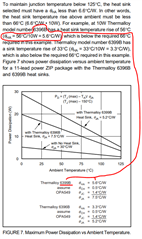Thanks!

• Hello Delany,

When the OPA549 datasheet was written more than 20 years ago the 6396B and 6399B heatsinks where produced by Thermalloy. It looks like the company has changed hands and name a few times, but the heatsinks are still being produced under the original model numbers.

The theta ϴHA numbers you mention from the OPA549 datasheet section represent 5.6 °C/W for the 6396B, and 3.3 °C/W for the 6399B, respectively. The 6399B is physically larger than the 6396B and has more surface area, which results in lower thermal resistance.

A more complete picture of the ϴHA performance of the two hear sinks can be found in their Boyd-AAVID datasheet. The ϴHA information is on the right hand vertical axis. OHA can be determined more precisely relative to power dissipation and air flow rate.

https://www.boydcorp.com/aavid-datasheets/Board-Level-Cooling-High-Power-Extruded-6390.pdf

Regards, Thomas

Precision Amplifiers Applications Engineering

• Hi Delaney,

As Thomas pointed out, the heat sink to ambient figures and part numbers have changed.

If you provide us with power dissipated heat Pd in OPA549,  Ta operating temperature (worst case) and air flow rate across the heat sink or static etc., we may be able to calculate some of heat sink requirements for you. Normally, larger the heatsink, more dissipated surface area, higher heat conduction material etc. will have lower figure in Heat-Sink-To-Ambient (C/W), which is more efficient heat sink in cooling, and it will keep OPA549 cooler for a given application.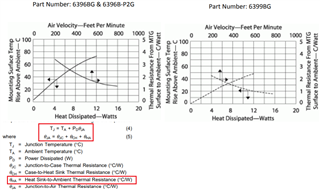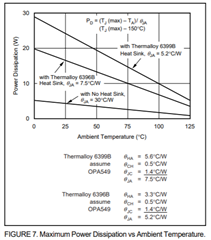If you need additional help, please let us know.

Best,

Raymond

• Hi Raymond,

Thank you!

Ambient temp would be 28C max, Peak power ~14W. Calculated heatsink with ThetaHA = 5.2C/W, does this sound about right?

Best,

Delaney

• Hi Delaney,

I do not have all the information to calculate ThetaHA. Pd power may not be the peak power. The Pd or dissipated power is a function of supplied voltage, output voltage and load current that goes through the internal die of OPA592. The peak power here may refer to the max. wattage of a load. I need to know the delta voltage from Vcc-Vout and load current that goes through the internal OPA592 for a worst case in order to calculate Pd, which is not necessarily equal to a load's peak power.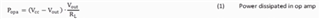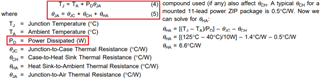Enclosed is a captured image that may help you to calculate Pd at OPA592.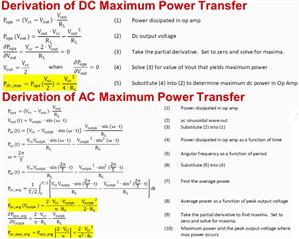If you have additional questions, please let us know.

Raymond

•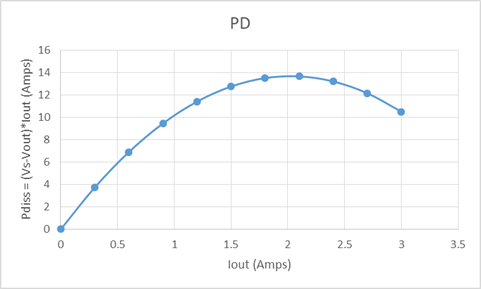Does this graph have the information I would need?

• Hi Delaney,

Yes, this is the plot for AC input. You provided 14W in peak power, I need to know what is load current and OPA549's output peak  voltage. From the figures, you are able to calculate Pd = (Vcc - Vout)Iout_max or use the following equations in AC.

From the graph, max. Pd is occurred at Iout approx. 2A or approx. Pd = 28W.14W peak power at output can come from high voltage &low output current, or medium voltage & current or low voltage & high load current etc. in (Vcc-Vout)*Iout products. Since I do not have these figures, I am unable to calculate Pd per your inquiry.

Best,

Raymond

• I see, thank you.

The load is an ~3.33ohm TEC

The power supply to the op-amp is 13.5V (This is generated from LMZM33604RLXR)

below is Vout vs. Iout plot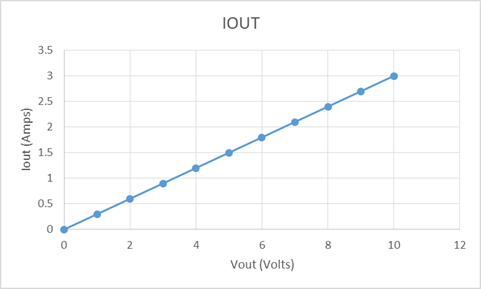I will do the calculations and hopefully it matches the calculated heatsink with ThetaHA = 5.2C/W.• Hi Delaney,

Since OPA549 is used for TEC application, the part's output is operating in DC voltage to cool or heat TEC for temperature control.

With 14W at the output, R_load = 3.33Ω, the OPA549's output voltage is regulated at V^2/R_load = 14W or Vout = 6.83Vdc and output current is 14W=(I^2)*R_load or Iout = 2.05Adc

Since Vcc = 13.5C, Vcc-Vout = 13.5V - 6.8V = 6.7Vdc. Pd = 6.83V * 2.05A = 14 W. And your ThetaHA calculation is correct.

Best,

Raymond

• Thanks so much for the help Raymond!